Next: Amplifier Model Up: Passive RCL Filters Previous: Passive RCL Filters

## Series RCL Circuit

Consider the RCL circuits as shown in figure 3.9. Each has a low-frequency and high-frequency approximation. Considering the band-reject filter (figure 3.6d) we obtain for the transfer function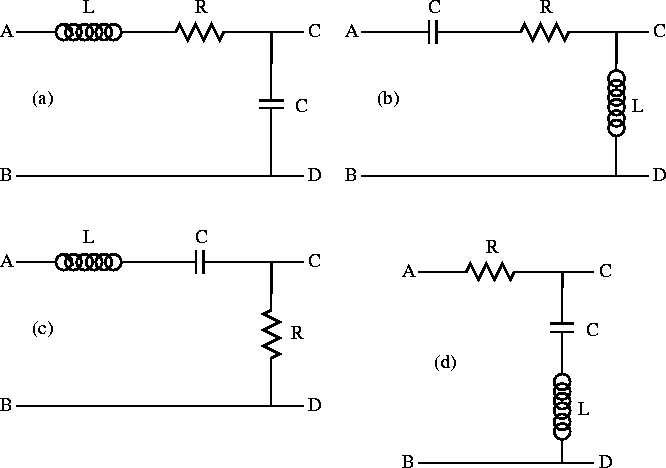Figure 3.9:  LCR filters: a) low-pass, b) high-pass, c) band-pass and d) band-reject.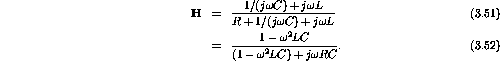The approximations are: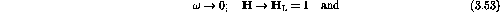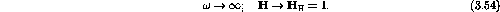We notice a zero in the transfer function at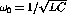. In the low-medium frequency range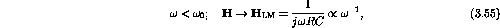for high-medium frequencies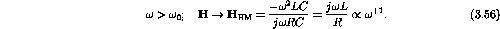Solving for the corner frequencies we have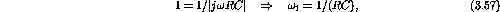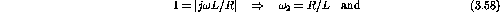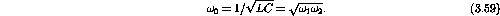Example: Sketch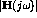for the LCR circuit shown in figure 3.10 for the two conditions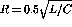and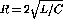. In each case, determine the values of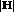at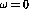,, and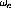, and label these points on the sketches.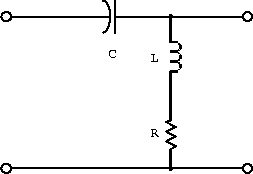Figure 3.10:  LCR circuit with two components across the output.

The transfer function is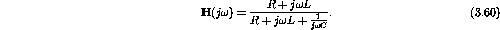Forsmall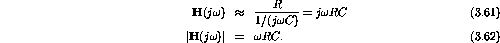For large: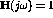.

For the corner frequency: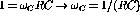.

For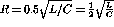,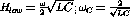.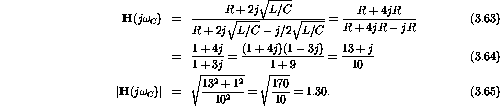For,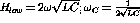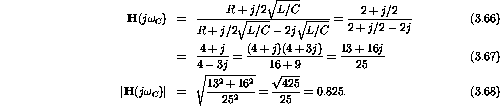Figure 3.11 is a sketch of the transfer functions.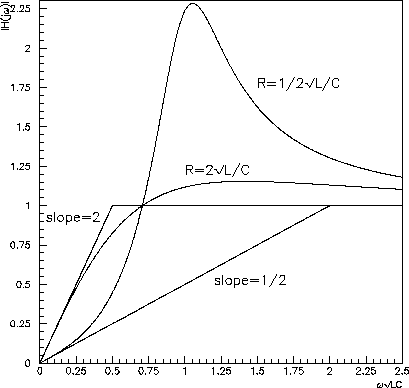Figure 3.11:  Sketch of the transfer functions for the above circuit.

Example:
1. Write an expression for the transfer function of the circuit shown in figure 3.12.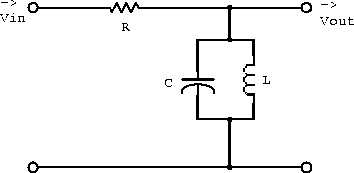Figure 3.12:  Circuit with components in parallel at the output.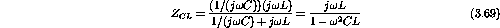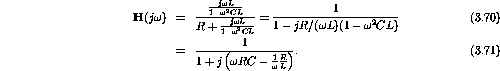2. What phase shift is introduced by this filter at very small and very large frequencies?

For large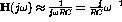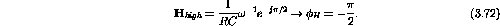For small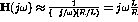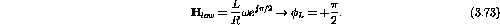3. On a log-log scale, sketchand the phase shift as a function of.

For the corner frequency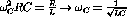.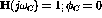.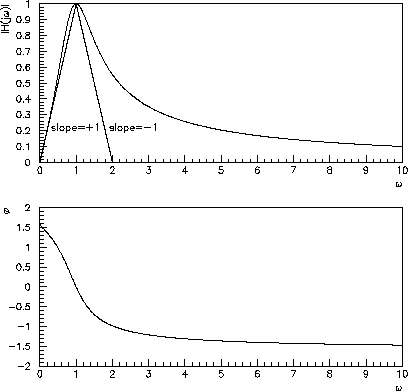Figure 3.13: Transfer function and phase shift for the above circuit.

Doug Gingrich
Tue Jul 13 16:55:15 EDT 1999